# Selina Solutions Concise Mathematics Class 6 Chapter 18: Fundamental Concepts

Selina Solutions Concise Mathematics Class 6 Chapter 18 Fundamental Concepts has detailed answers formulated by highly experienced teachers at BYJU’S. Students who aim to come out with flying colours in the examination, are suggested to practice exercise wise problem solutions repeatedly. Selina solutions, prepared as per the current ICSE exam, help students clear their doubts quickly. Solutions PDF are a key source among students, in boosting exam preparation. Students can use Selina Solutions Concise Mathematics Class 6 Chapter 18 Fundamental Concepts PDF, from the below links, whenever necessary

This chapter explains the elementary treatment and operation on various terms with suitable examples. The Solution PDF has answers for each and every question in simple language, as per the student’s understanding capacity.

## Selina Solutions Concise Mathematics Class 6 Chapter 18 Fundamental Concepts Download PDF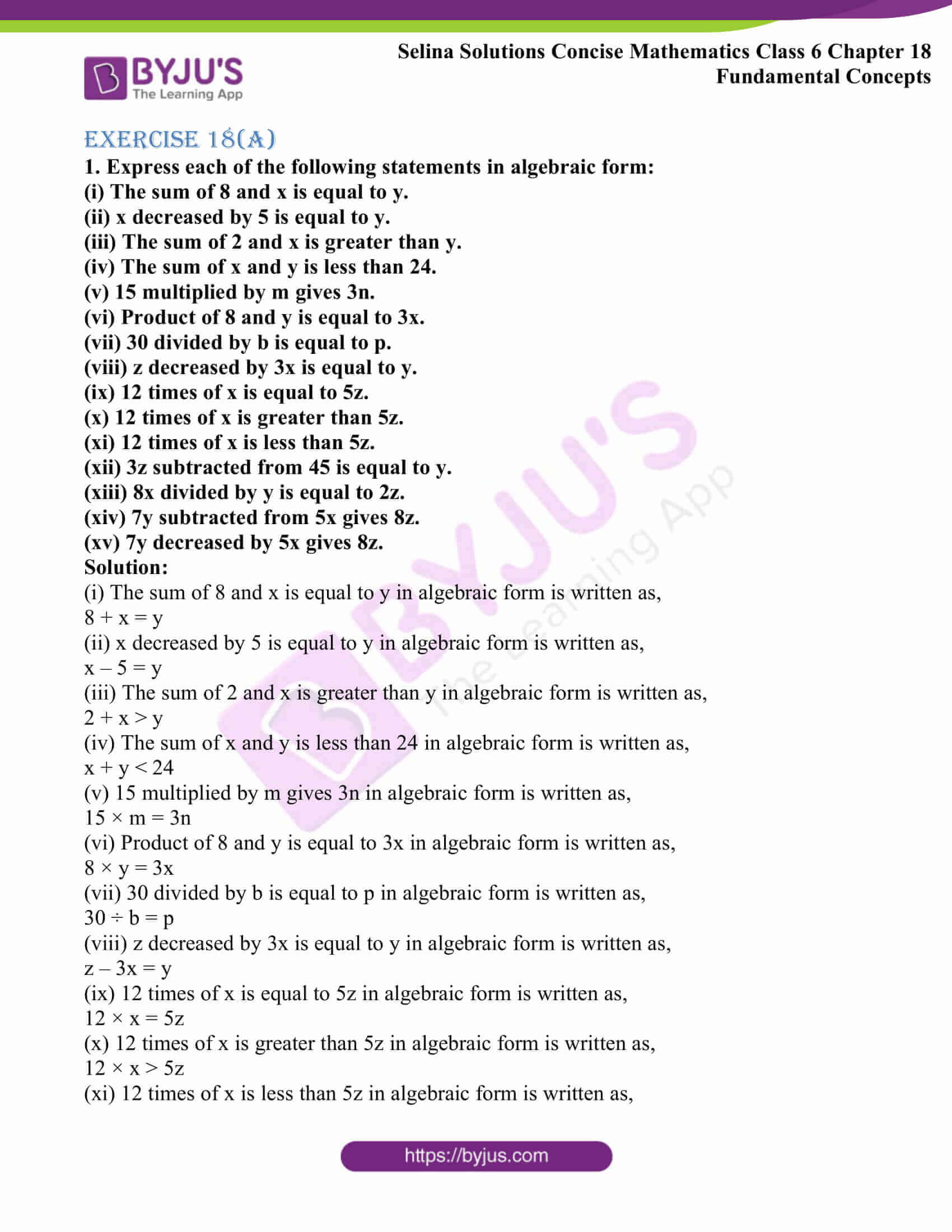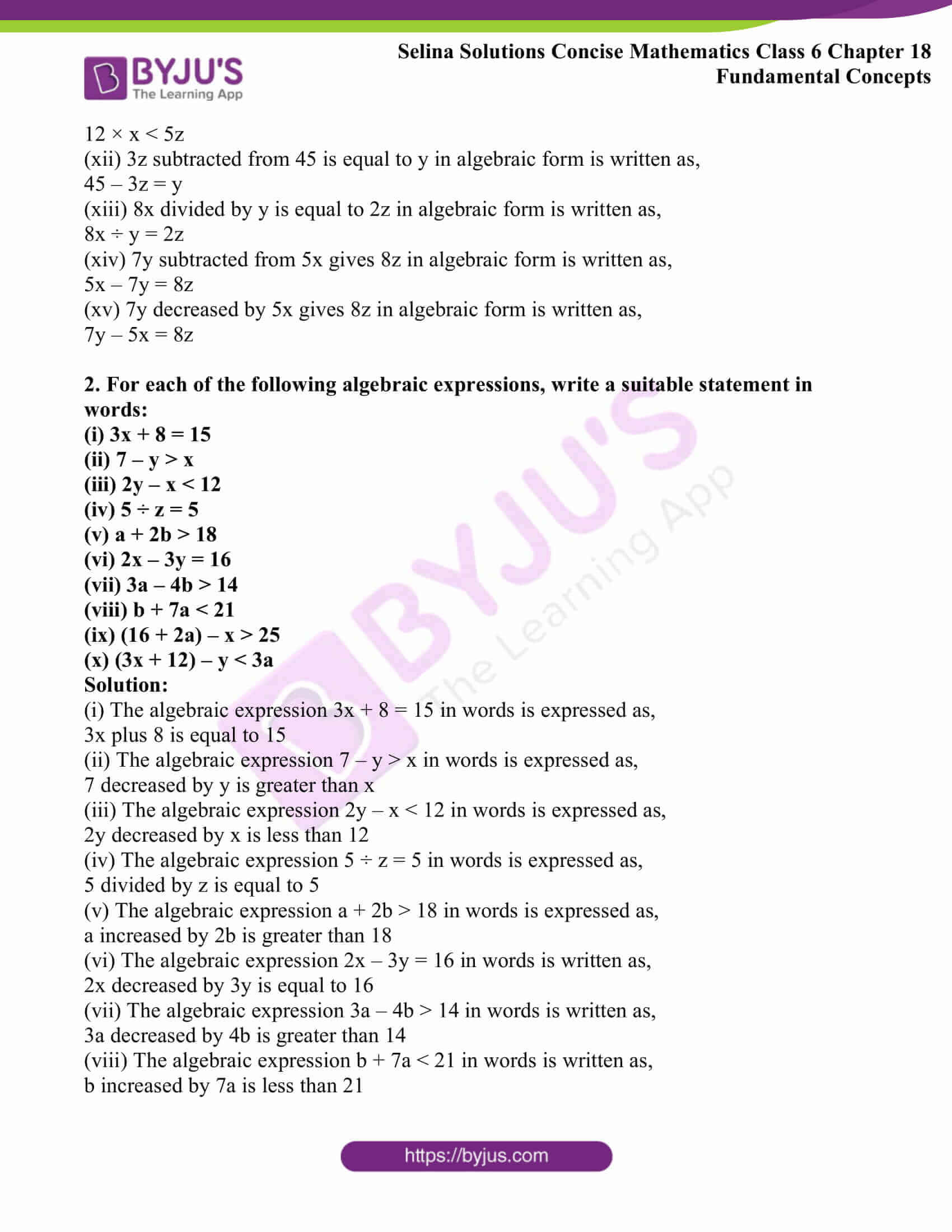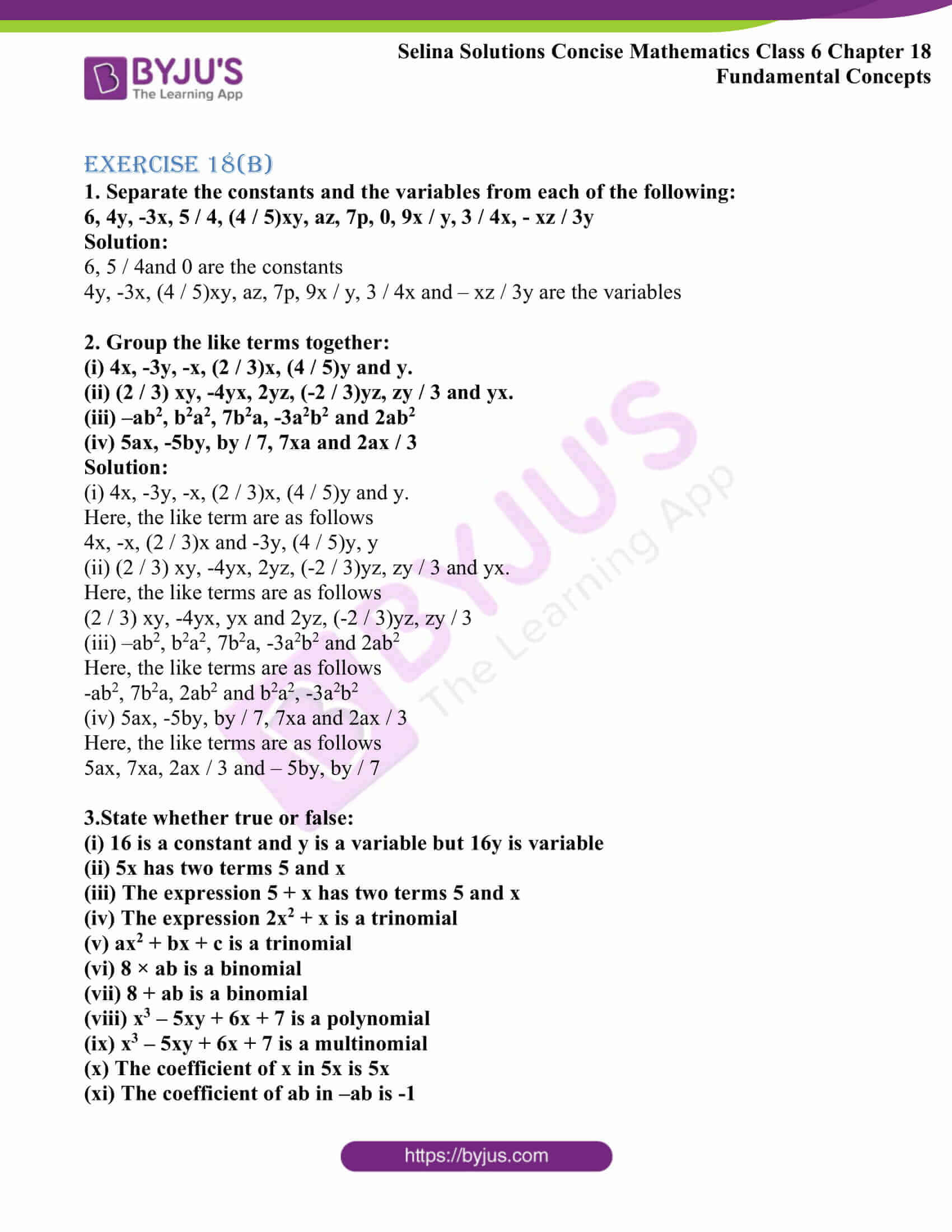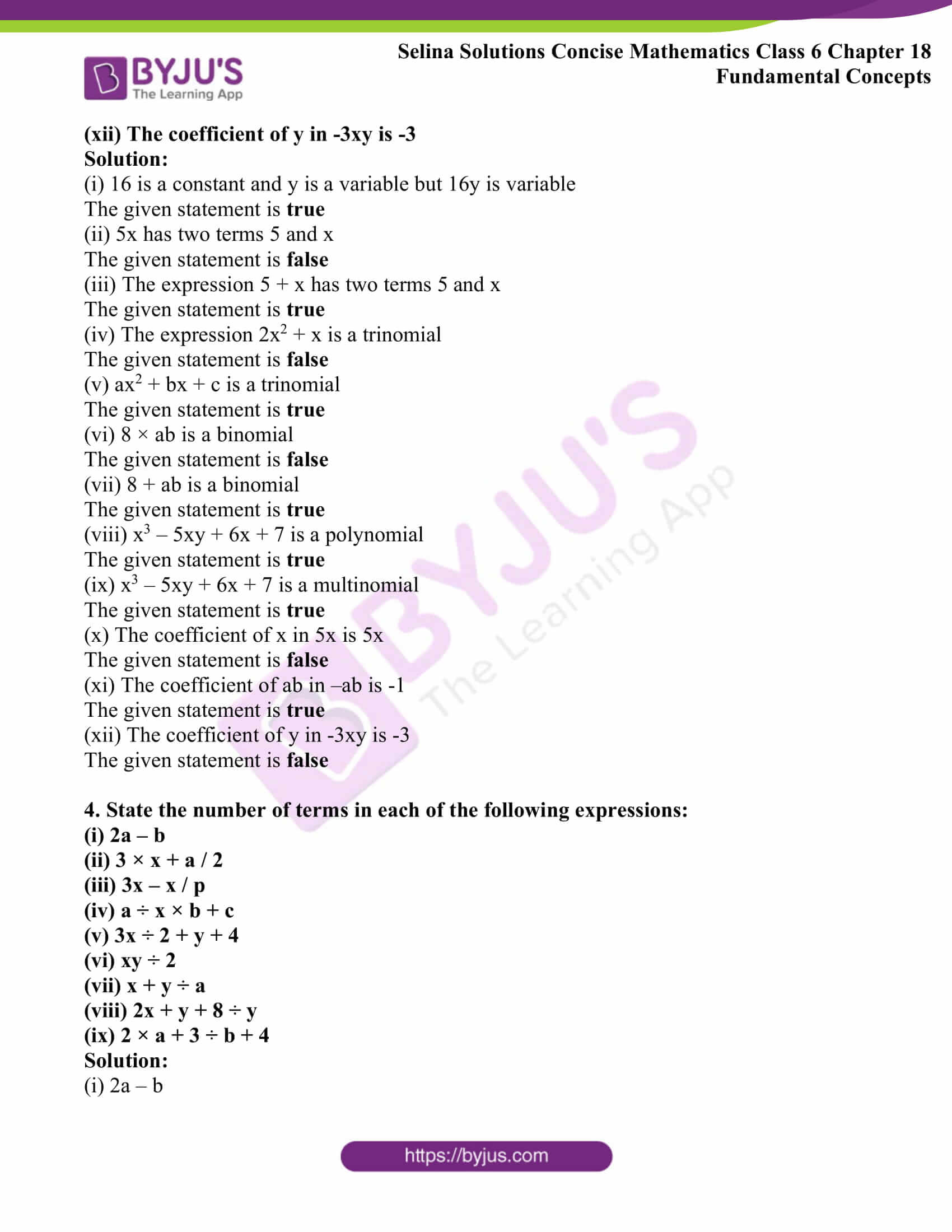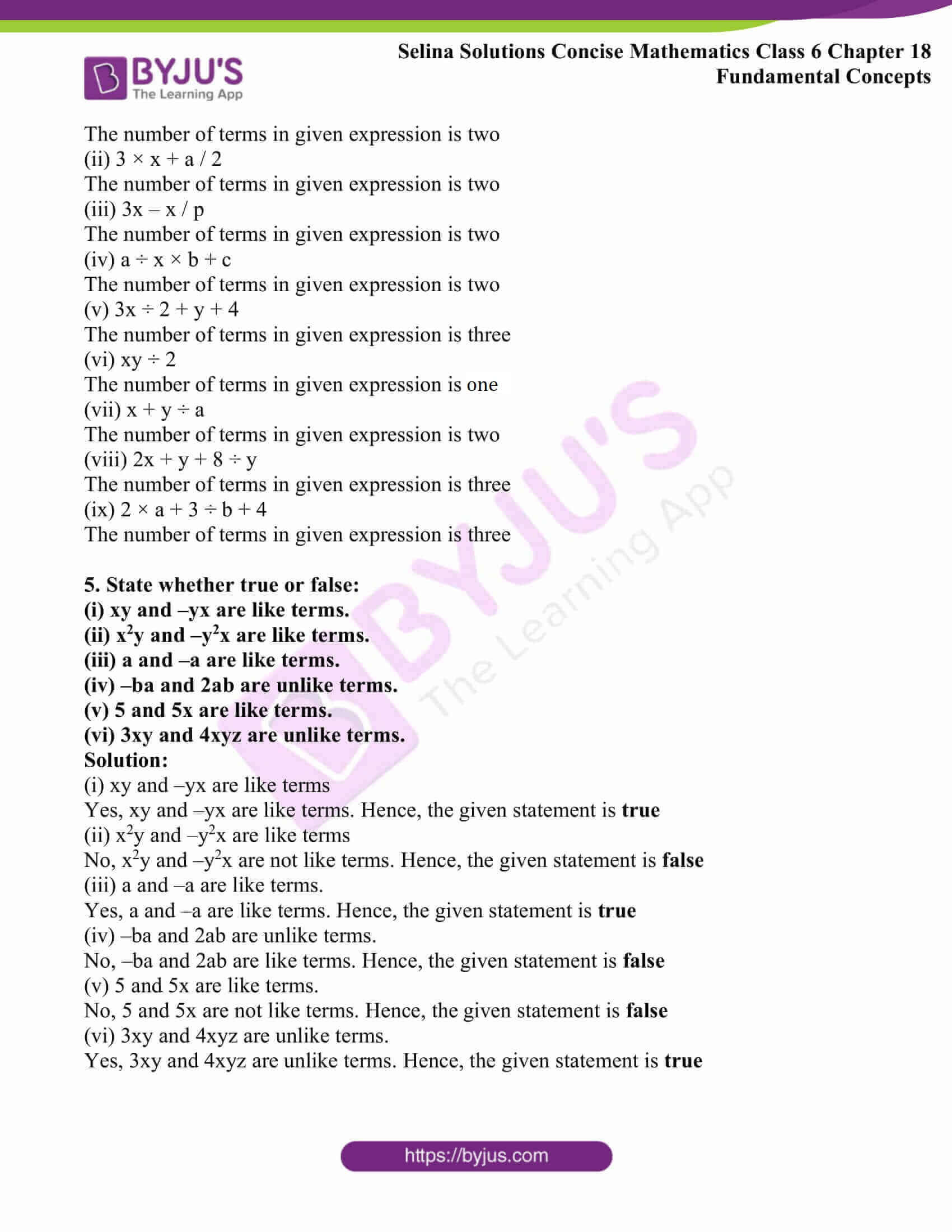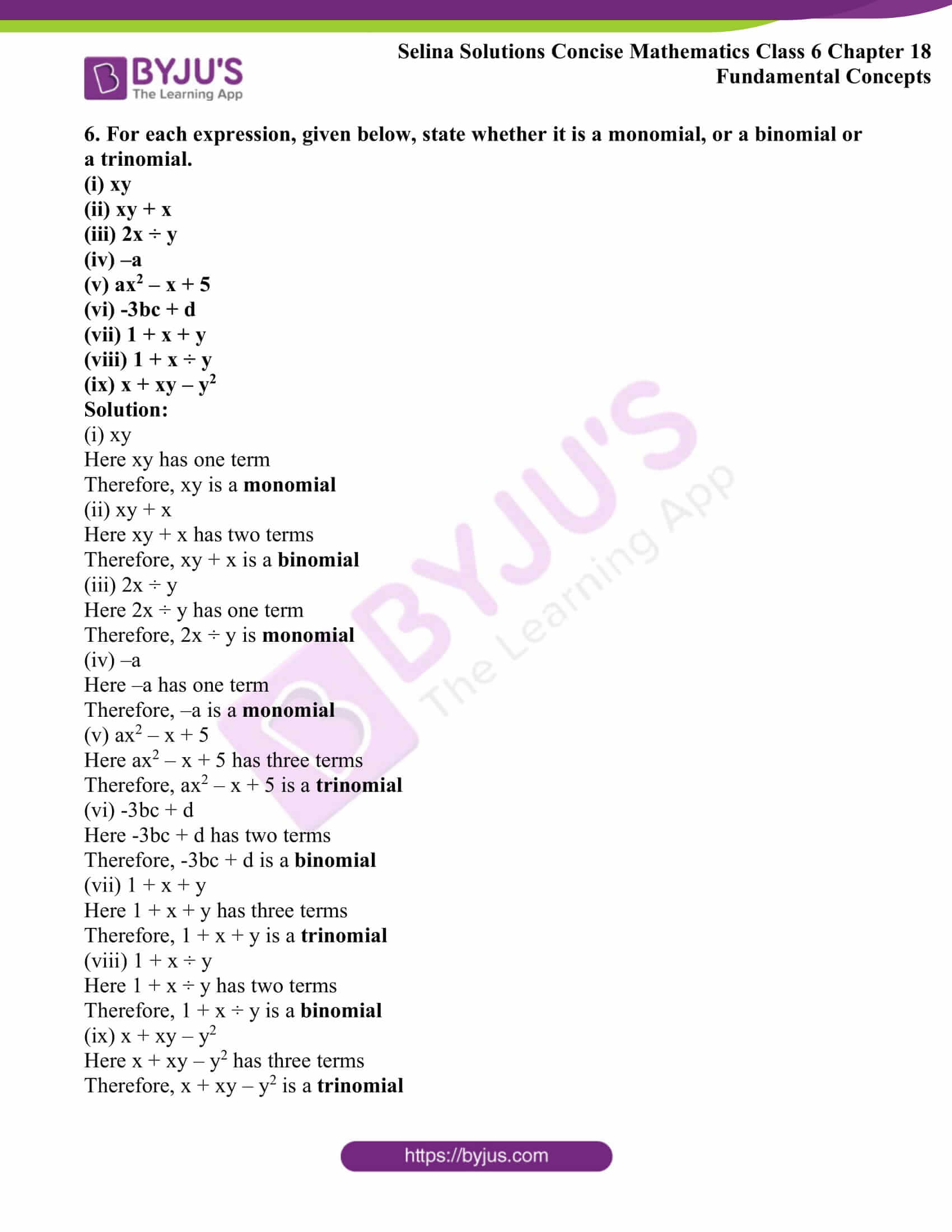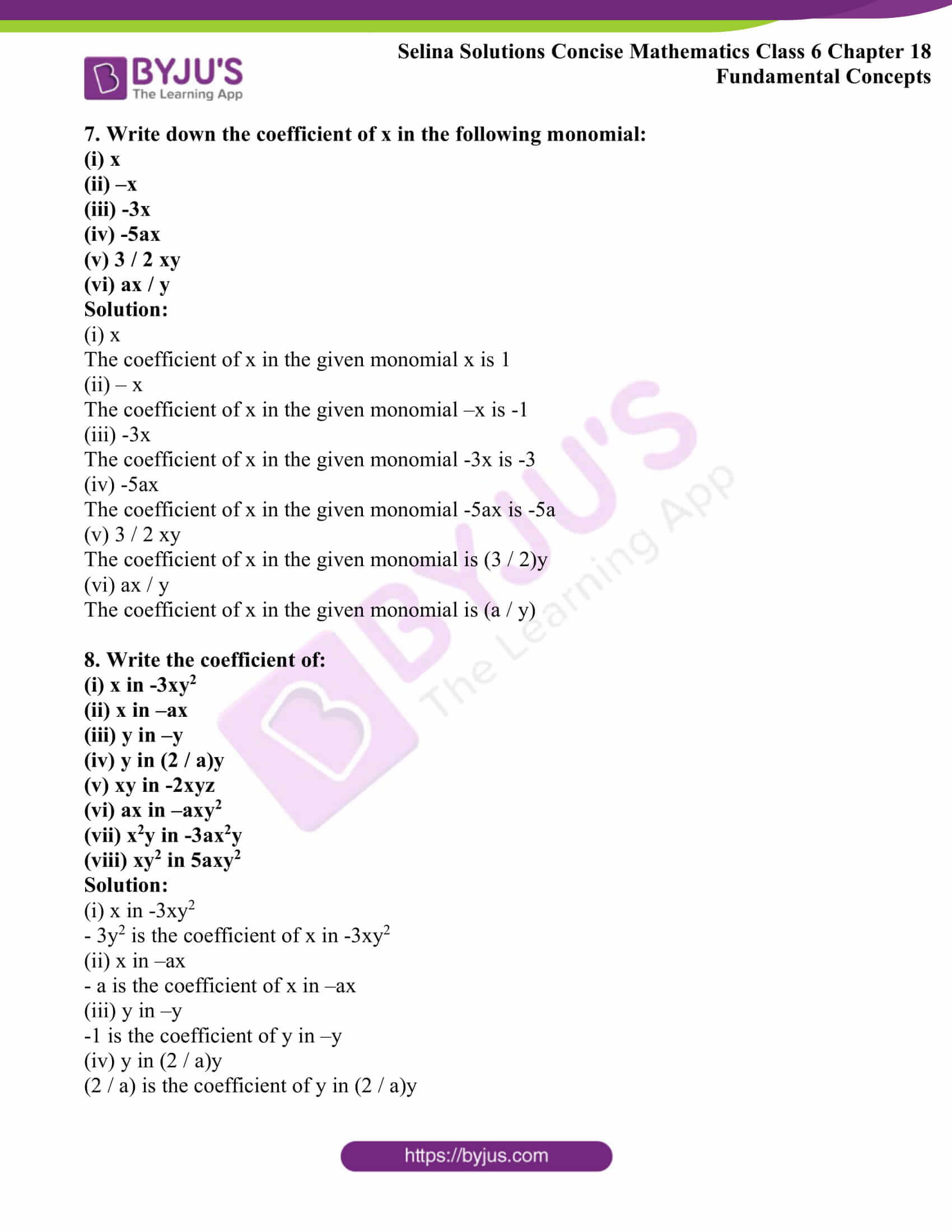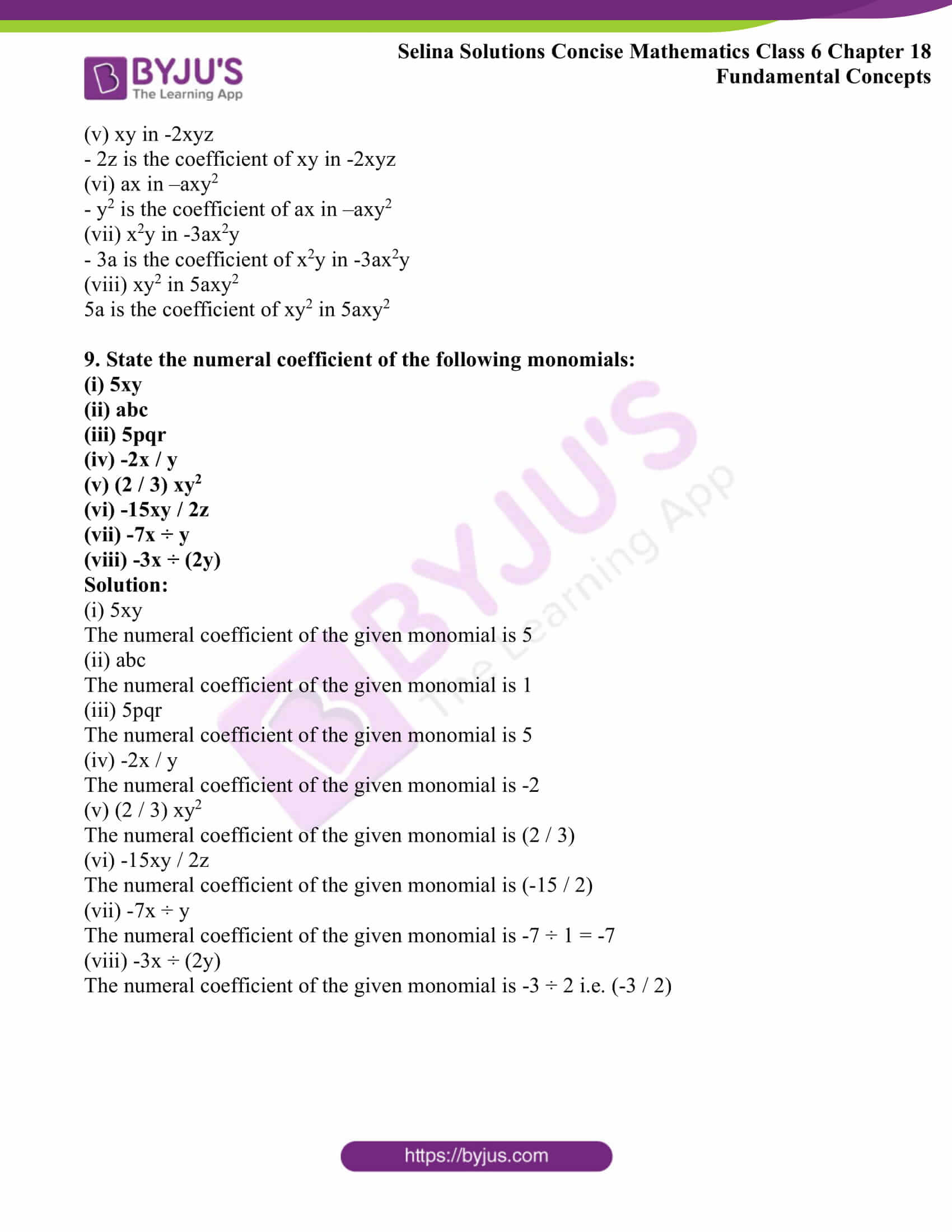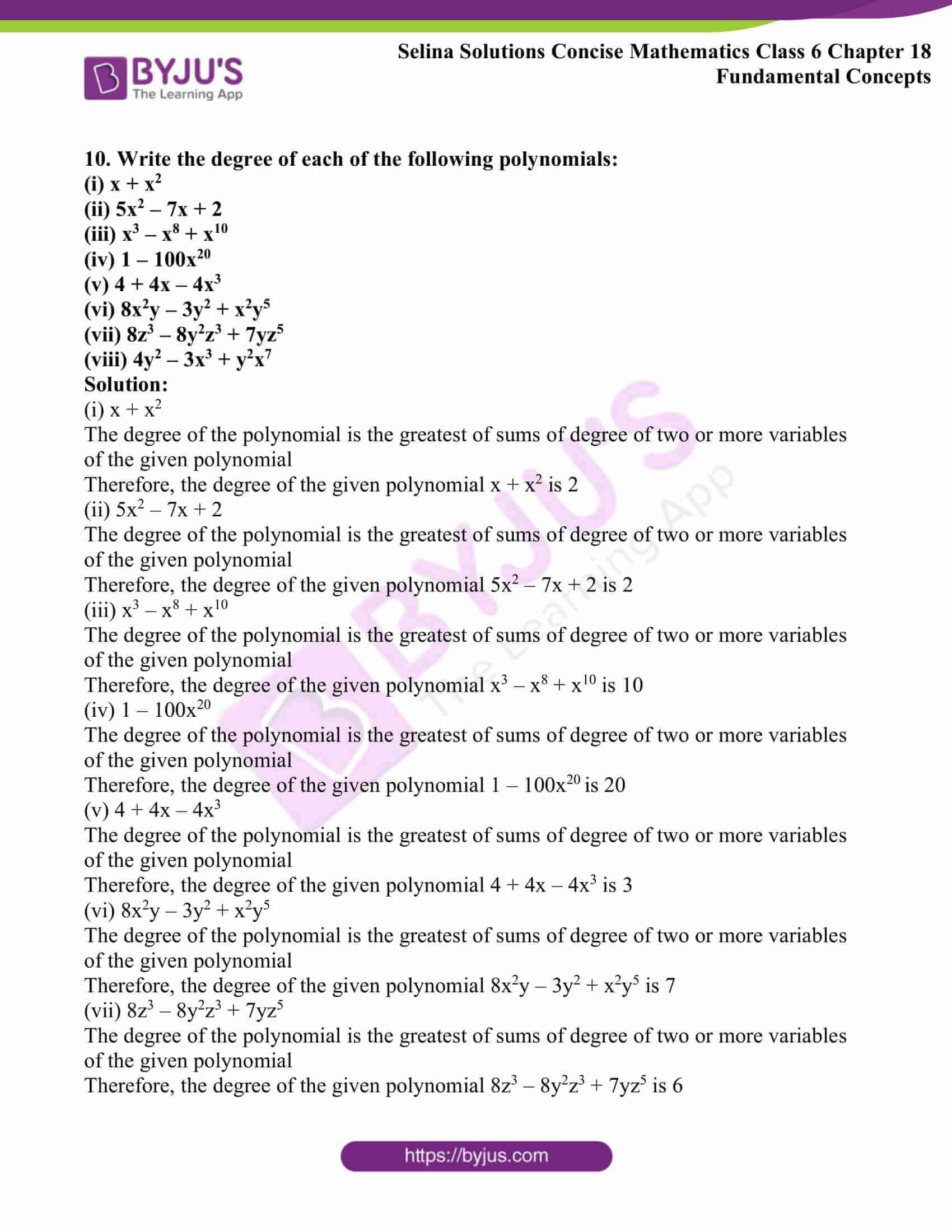### Exercises of Selina Solutions Concise Mathematics Class 6 Chapter 18: Fundamental Concepts

Exercise 18(A) Solutions

Exercise 18(B) Solutions

## Access Selina Solutions Concise Mathematics Class 6 Chapter 18: Fundamental Concepts

Exercise 18(A)

1. Express each of the following statements in algebraic form:

(i) The sum of 8 and x is equal to y.

(ii) x decreased by 5 is equal to y.

(iii) The sum of 2 and x is greater than y.

(iv) The sum of x and y is less than 24.

(v) 15 multiplied by m gives 3n.

(vi) Product of 8 and y is equal to 3x.

(vii) 30 divided by b is equal to p.

(viii) z decreased by 3x is equal to y.

(ix) 12 times of x is equal to 5z.

(x) 12 times of x is greater than 5z.

(xi) 12 times of x is less than 5z.

(xii) 3z subtracted from 45 is equal to y.

(xiii) 8x divided by y is equal to 2z.

(xiv) 7y subtracted from 5x gives 8z.

(xv) 7y decreased by 5x gives 8z.

Solution:

(i) The sum of 8 and x is equal to y in algebraic form is written as,

8 + x = y

(ii) x decreased by 5 is equal to y in algebraic form is written as,

x – 5 = y

(iii) The sum of 2 and x is greater than y in algebraic form is written as,

2 + x > y

(iv) The sum of x and y is less than 24 in algebraic form is written as,

x + y < 24

(v) 15 multiplied by m gives 3n in algebraic form is written as,

15 × m = 3n

(vi) Product of 8 and y is equal to 3x in algebraic form is written as,

8 × y = 3x

(vii) 30 divided by b is equal to p in algebraic form is written as,

30 ÷ b = p

(viii) z decreased by 3x is equal to y in algebraic form is written as,

z – 3x = y

(ix) 12 times of x is equal to 5z in algebraic form is written as,

12 × x = 5z

(x) 12 times of x is greater than 5z in algebraic form is written as,

12 × x > 5z

(xi) 12 times of x is less than 5z in algebraic form is written as,

12 × x < 5z

(xii) 3z subtracted from 45 is equal to y in algebraic form is written as,

45 – 3z = y

(xiii) 8x divided by y is equal to 2z in algebraic form is written as,

8x ÷ y = 2z

(xiv) 7y subtracted from 5x gives 8z in algebraic form is written as,

5x – 7y = 8z

(xv) 7y decreased by 5x gives 8z in algebraic form is written as,

7y – 5x = 8z

2. For each of the following algebraic expressions, write a suitable statement in words:

(i) 3x + 8 = 15

(ii) 7 – y > x

(iii) 2y – x < 12

(iv) 5 ÷ z = 5

(v) a + 2b > 18

(vi) 2x – 3y = 16

(vii) 3a – 4b > 14

(viii) b + 7a < 21

(ix) (16 + 2a) – x > 25

(x) (3x + 12) – y < 3a

Solution:

(i) The algebraic expression 3x + 8 = 15 in words is expressed as,

3x plus 8 is equal to 15

(ii) The algebraic expression 7 – y > x in words is expressed as,

7 decreased by y is greater than x

(iii) The algebraic expression 2y – x < 12 in words is expressed as,

2y decreased by x is less than 12

(iv) The algebraic expression 5 ÷ z = 5 in words is expressed as,

5 divided by z is equal to 5

(v) The algebraic expression a + 2b > 18 in words is expressed as,

a increased by 2b is greater than 18

(vi) The algebraic expression 2x – 3y = 16 in words is written as,

2x decreased by 3y is equal to 16

(vii) The algebraic expression 3a – 4b > 14 in words is written as,

3a decreased by 4b is greater than 14

(viii) The algebraic expression b + 7a < 21 in words is written as,

b increased by 7a is less than 21

(ix) The algebraic expression (16 + 2a) – x > 25 in words is written as,

The sum of 16 and 2a decreased by x is greater than 25

(x) The algebraic expression (3x + 12) – y < 3a in words is written as,

The sum of 3x and 12 decreased by y is less than 3a

Exercise 18(B)

1. Separate the constants and the variables from each of the following:

6, 4y, -3x, 5 / 4, (4 / 5)xy, az, 7p, 0, 9x / y, 3 / 4x, – xz / 3y

Solution:

6, 5 / 4and 0 are the constants

4y, -3x, (4 / 5)xy, az, 7p, 9x / y, 3 / 4x and – xz / 3y are the variables

2. Group the like terms together:

(i) 4x, -3y, -x, (2 / 3)x, (4 / 5)y and y.

(ii) (2 / 3) xy, -4yx, 2yz, (-2 / 3)yz, zy / 3 and yx.

(iii) –ab2, b2a2, 7b2a, -3a2b2 and 2ab2

(iv) 5ax, -5by, by / 7, 7xa and 2ax / 3

Solution:

(i) 4x, -3y, -x, (2 / 3)x, (4 / 5)y and y.

Here, the like term are as follows

4x, -x, (2 / 3)x and -3y, (4 / 5)y, y

(ii) (2 / 3) xy, -4yx, 2yz, (-2 / 3)yz, zy / 3 and yx.

Here, the like terms are as follows

(2 / 3) xy, -4yx, yx and 2yz, (-2 / 3)yz, zy / 3

(iii) –ab2, b2a2, 7b2a, -3a2b2 and 2ab2

Here, the like terms are as follows

-ab2, 7b2a, 2ab2 and b2a2, -3a2b2

(iv) 5ax, -5by, by / 7, 7xa and 2ax / 3

Here, the like terms are as follows

5ax, 7xa, 2ax / 3 and – 5by, by / 7

3.State whether true or false:

(i) 16 is a constant and y is a variable but 16y is variable

(ii) 5x has two terms 5 and x

(iii) The expression 5 + x has two terms 5 and x

(iv) The expression 2x2 + x is a trinomial

(v) ax2 + bx + c is a trinomial

(vi) 8 × ab is a binomial

(vii) 8 + ab is a binomial

(viii) x3 – 5xy + 6x + 7 is a polynomial

(ix) x3 – 5xy + 6x + 7 is a multinomial

(x) The coefficient of x in 5x is 5x

(xi) The coefficient of ab in –ab is -1

(xii) The coefficient of y in -3xy is -3

Solution:

(i) 16 is a constant and y is a variable but 16y is variable

The given statement is true

(ii) 5x has two terms 5 and x

The given statement is false

(iii) The expression 5 + x has two terms 5 and x

The given statement is true

(iv) The expression 2x2 + x is a trinomial

The given statement is false

(v) ax2 + bx + c is a trinomial

The given statement is true

(vi) 8 × ab is a binomial

The given statement is false

(vii) 8 + ab is a binomial

The given statement is true

(viii) x3 – 5xy + 6x + 7 is a polynomial

The given statement is true

(ix) x3 – 5xy + 6x + 7 is a multinomial

The given statement is true

(x) The coefficient of x in 5x is 5x

The given statement is false

(xi) The coefficient of ab in –ab is -1

The given statement is true

(xii) The coefficient of y in -3xy is -3

The given statement is false

4. State the number of terms in each of the following expressions:

(i) 2a – b

(ii) 3 × x + a / 2

(iii) 3x – x / p

(iv) a ÷ x × b + c

(v) 3x ÷ 2 + y + 4

(vi) xy ÷ 2

(vii) x + y ÷ a

(viii) 2x + y + 8 ÷ y

(ix) 2 × a + 3 ÷ b + 4

Solution:

(i) 2a – b

The number of terms in given expression is two

(ii) 3 × x + a / 2

The number of terms in given expression is two

(iii) 3x – x / p

The number of terms in given expression is two

(iv) a ÷ x × b + c

The number of terms in given expression is two

(v) 3x ÷ 2 + y + 4

The number of terms in given expression is three

(vi) xy ÷ 2

The number of terms in given expression is one

(vii) x + y ÷ a

The number of terms in given expression is two

(viii) 2x + y + 8 ÷ y

The number of terms in given expression is three

(ix) 2 × a + 3 ÷ b + 4

The number of terms in given expression is three

5. State whether true or false:

(i) xy and –yx are like terms.

(ii) x2y and –y2x are like terms.

(iii) a and –a are like terms.

(iv) –ba and 2ab are unlike terms.

(v) 5 and 5x are like terms.

(vi) 3xy and 4xyz are unlike terms.

Solution:

(i) xy and –yx are like terms

Yes, xy and –yx are like terms. Hence, the given statement is true

(ii) x2y and –y2x are like terms

No, x2y and –y2x are not like terms. Hence, the given statement is false

(iii) a and –a are like terms.

Yes, a and –a are like terms. Hence, the given statement is true

(iv) –ba and 2ab are unlike terms.

No, –ba and 2ab are like terms. Hence, the given statement is false

(v) 5 and 5x are like terms.

No, 5 and 5x are not like terms. Hence, the given statement is false

(vi) 3xy and 4xyz are unlike terms.

Yes, 3xy and 4xyz are unlike terms. Hence, the given statement is true

6. For each expression, given below, state whether it is a monomial, or a binomial or a trinomial.

(i) xy

(ii) xy + x

(iii) 2x ÷ y

(iv) –a

(v) ax2 – x + 5

(vi) -3bc + d

(vii) 1 + x + y

(viii) 1 + x ÷ y

(ix) x + xy – y2

Solution:

(i) xy

Here xy has one term

Therefore, xy is a monomial

(ii) xy + x

Here xy + x has two terms

Therefore, xy + x is a binomial

(iii) 2x ÷ y

Here 2x ÷ y has one term

Therefore, 2x ÷ y is monomial

(iv) –a

Here –a has one term

Therefore, –a is a monomial

(v) ax2 – x + 5

Here ax2 – x + 5 has three terms

Therefore, ax2 – x + 5 is a trinomial

(vi) -3bc + d

Here -3bc + d has two terms

Therefore, -3bc + d is a binomial

(vii) 1 + x + y

Here 1 + x + y has three terms

Therefore, 1 + x + y is a trinomial

(viii) 1 + x ÷ y

Here 1 + x ÷ y has two terms

Therefore, 1 + x ÷ y is a binomial

(ix) x + xy – y2

Here x + xy – y2 has three terms

Therefore, x + xy – y2 is a trinomial

7. Write down the coefficient of x in the following monomial:

(i) x

(ii) –x

(iii) -3x

(iv) -5ax

(v) 3 / 2 xy

(vi) ax / y

Solution:

(i) x

The coefficient of x in the given monomial x is 1

(ii) – x

The coefficient of x in the given monomial –x is -1

(iii) -3x

The coefficient of x in the given monomial -3x is -3

(iv) -5ax

The coefficient of x in the given monomial -5ax is -5a

(v) 3 / 2 xy

The coefficient of x in the given monomial is (3 / 2)y

(vi) ax / y

The coefficient of x in the given monomial is (a / y)

8. Write the coefficient of:

(i) x in -3xy2

(ii) x in –ax

(iii) y in –y

(iv) y in (2 / a)y

(v) xy in -2xyz

(vi) ax in –axy2

(vii) x2y in -3ax2y

(viii) xy2 in 5axy2

Solution:

(i) x in -3xy2

– 3y2 is the coefficient of x in -3xy2

(ii) x in –ax

– a is the coefficient of x in –ax

(iii) y in –y

-1 is the coefficient of y in –y

(iv) y in (2 / a)y

(2 / a) is the coefficient of y in (2 / a)y

(v) xy in -2xyz

– 2z is the coefficient of xy in -2xyz

(vi) ax in –axy2

– y2 is the coefficient of ax in –axy2

(vii) x2y in -3ax2y

– 3a is the coefficient of x2y in -3ax2y

(viii) xy2 in 5axy2

5a is the coefficient of xy2 in 5axy2

9. State the numeral coefficient of the following monomials:

(i) 5xy

(ii) abc

(iii) 5pqr

(iv) -2x / y

(v) (2 / 3) xy2

(vi) -15xy / 2z

(vii) -7x ÷ y

(viii) -3x ÷ (2y)

Solution:

(i) 5xy

The numeral coefficient of the given monomial is 5

(ii) abc

The numeral coefficient of the given monomial is 1

(iii) 5pqr

The numeral coefficient of the given monomial is 5

(iv) -2x / y

The numeral coefficient of the given monomial is -2

(v) (2 / 3) xy2

The numeral coefficient of the given monomial is (2 / 3)

(vi) -15xy / 2z

The numeral coefficient of the given monomial is (-15 / 2)

(vii) -7x ÷ y

The numeral coefficient of the given monomial is -7 ÷ 1 = -7

(viii) -3x ÷ (2y)

The numeral coefficient of the given monomial is -3 ÷ 2 i.e. (-3 / 2)

10. Write the degree of each of the following polynomials:

(i) x + x2

(ii) 5x2 – 7x + 2

(iii) x3 – x8 + x10

(iv) 1 – 100x20

(v) 4 + 4x – 4x3

(vi) 8x2y – 3y2 + x2y5

(vii) 8z3 – 8y2z3 + 7yz5

(viii) 4y2 – 3x3 + y2x7

Solution:

(i) x + x2

The degree of the polynomial is the greatest of sums of degree of two or more variables of the given polynomial

Therefore, the degree of the given polynomial x + x2 is 2

(ii) 5x2 – 7x + 2

The degree of the polynomial is the greatest of sums of degree of two or more variables of the given polynomial

Therefore, the degree of the given polynomial 5x2 – 7x + 2 is 2

(iii) x3 – x8 + x10

The degree of the polynomial is the greatest of sums of degree of two or more variables of the given polynomial

Therefore, the degree of the given polynomial x3 – x8 + x10 is 10

(iv) 1 – 100x20

The degree of the polynomial is the greatest of sums of degree of two or more variables of the given polynomial

Therefore, the degree of the given polynomial 1 – 100x20 is 20

(v) 4 + 4x – 4x3

The degree of the polynomial is the greatest of sums of degree of two or more variables of the given polynomial

Therefore, the degree of the given polynomial 4 + 4x – 4x3 is 3

(vi) 8x2y – 3y2 + x2y5

The degree of the polynomial is the greatest of sums of degree of two or more variables of the given polynomial

Therefore, the degree of the given polynomial 8x2y – 3y2 + x2y5 is 7

(vii) 8z3 – 8y2z3 + 7yz5

The degree of the polynomial is the greatest of sums of degree of two or more variables of the given polynomial

Therefore, the degree of the given polynomial 8z3 – 8y2z3 + 7yz5 is 6

(viii) 4y2 – 3x3 + y2x7

The degree of the polynomial is the greatest of sums of degree of two or more variables of the given polynomial

Therefore, the degree of the given polynomial 4y2 – 3x3 + y2x7 is 9